﻿ 电梯运行方案的计算机模拟优化设计 Computer Simulation and Optimization Design of Elevator Operation Plan

Modeling and Simulation
Vol. 07  No. 04 ( 2018 ), Article ID: 27786 , 14 pages
10.12677/MOS.2018.74025

Computer Simulation and Optimization Design of Elevator Operation Plan

Maoyi Zhang

School of Information and Control Engineering, China University of Mining and Technology, Xuzhou Jiangsu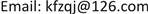Received: Nov. 9th, 2018; accepted: Nov. 21st, 2018; published: Nov. 29th, 2018ABSTRACT

This paper solves the design problem of elevator dispatching scheme in hospital outpatient building by computer simulation. This paper analyzes the following three problems: the use of elevators, the transportation efficiency in rush hours, and the complex elevator dispatching problem. This topic combines MATLAB software, mainly using the computer simulation method. The characteristic of this paper is to simulate the running process of the elevator with greater randomness, which greatly reduces the difficulty of solving the problem. This paper not only evaluates the existing elevator operation scheme, but also gives a better design for the elevator operation management scheme in high-rise commercial building, and provides a theoretical basis for it.

Keywords:Elevator, Dispatching, MATLAB1. 引言

2. 电梯调度问题提出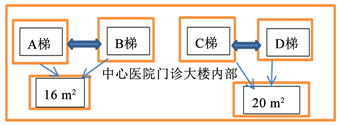Figure 1. Floor plan of the outpatient building of Xuzhou Central Hospital

3. 模型假设

4. 符号说明

${T}_{w}$ ：人均等电梯时间；

${\stackrel{¯}{T}}_{w}$ ：大规模仿真的平均人均等电梯时间；

$N$ ：停靠次数；

$\stackrel{¯}{N}$ ：大规模仿真的平均停靠次数；

${f}_{W}\left({T}_{w}\right)$ ：人均等待电梯时间不满意度隶属值；

${f}_{N}\left(N\right)$ ：平均停靠次数不满意度隶属值；

$\mu$ ：电梯承载率；

${T}_{End}$ ：该电梯当前批次的最终出发时间，也就是当前批次最后一个人的到达时间；

${L}_{End}$ ：该电梯当前批次的最大出发楼层，也就是电梯需要运行的最高位置，到达后即返回。

5. 概念引入及问题分析

5.1. 概念的引入

5.2. 问题分析

6. 模型的建立与求解

6.1. 模型准备与分析

6.1.1. 电梯停靠方案

6.1.2. 高峰时刻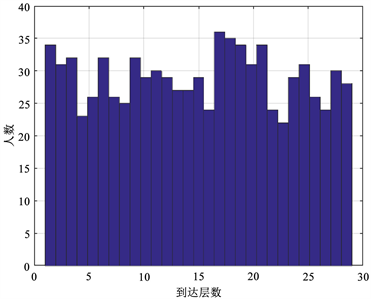(a)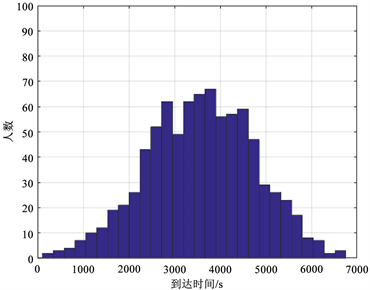(b)

Figure 2. Data distribution: (a) Arrival time distribution map; (b) Map of arrival levels

6.1.3. 电梯运行过程

Step 1：电梯开门，排在前面的一定数量人依次进入电梯，电梯门关闭，向上行驶；

Step 2：判断第i层是否有人下。若无，则不在第i层停靠，电梯驶向第i + 1层。若有，则电梯在第i层停靠，开门，要出人员依次走出电梯，关门。令i = i + 1，判断电梯是否达到最高期望楼层，若否向Step 2开头，若是则转向Step 3；

Step 3：电梯停靠在最高期望楼层，电梯中人员已全部下完，驶向靠在一层。

6.1.4. 方案设计思路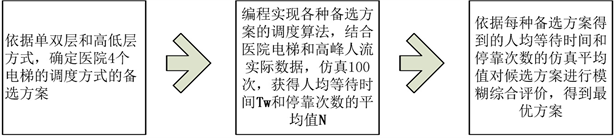Figure 3. Design flow chart of elevator dispatching plan

6.2. 问题1——电梯调度方案评价指标

6.2.1. 评价指标

$评价指标\left\{\begin{array}{cc}\begin{array}{l}满足乘客需求：\\ 节约能源：\end{array}& \begin{array}{l}平均等待时间\\ 停靠次数\end{array}\end{array}$

6.2.2. 电梯调度方案模糊综合评价方法

1) 平均等待指标的隶属化

$f\left(x\right)=\left\{\begin{array}{l}a{\left(x+b\right)}^{2},1\le x\le 3\\ c\mathrm{ln}x+d,3\le x\le 5\end{array}$

a) 当很满意时，隶属度为0，即 ${f}_{W}\left(200\right)=0$

b) 当较满意时，隶属度为0.8，即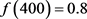c) 当很不满意时，隶属度为1，即 $f\left(1000\right)=1$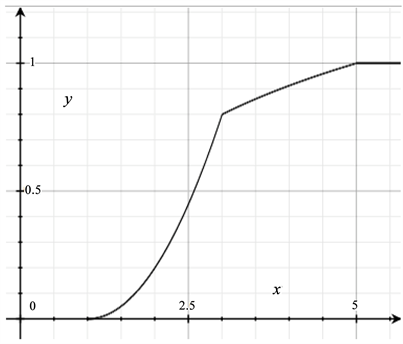Figure 4. Membership function of per capita waiting time

2) 停靠次数指标的隶属化

a) 当很满意时，隶属度为0，即f (125) = 0 ；

b) 当很不满意时，隶属度为1，即 $f\left(725\right)=1$Table 3. Depiction of the dissatisfaction of the number of stops

$f\left(x\right)=\left\{\begin{array}{cc}\begin{array}{l}0.25x-0.25,\\ 1,\end{array}& \begin{array}{l}1\le x\le 5\\ x>5\end{array}\end{array}$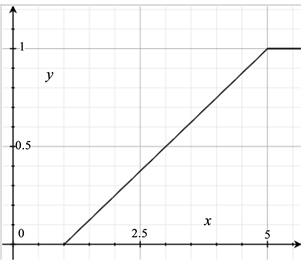Figure 5. Energy saving membership function

3) 线性加权评价模型

$f=0.8×{f}_{W}\left({T}_{w}\right)+0.2×{f}_{N}\left(N\right)$

$\left\{\begin{array}{l}{T}_{w}，{N}_{3}分别由计算机模拟数据整理得到\\ {f}_{W}\left({T}_{w}\right)=\left\{\begin{array}{cc}\begin{array}{l}0.2{\left(\frac{{T}_{w}}{200}-1\right)}^{2},\\ 0.3915\mathrm{ln}\frac{{T}_{w}}{200}+0.3699,\\ 1,\end{array}& \begin{array}{l}200\le x\le 600\\ 600\le x\le 1000\\ x>1000\end{array}\end{array}\\ {f}_{N}\left(N\right)=\left\{\begin{array}{cc}\begin{array}{l}0.25\frac{N}{125}-0.25,\\ 1,\end{array}& \begin{array}{l}125\le {T}_{a}\le 750\\ {T}_{a}>750\end{array}\end{array}\end{array}$

6.3. 问题2——电梯调度方案设计

6.3.1. 仿真统计思想

$\stackrel{¯}{N}=\frac{\underset{i=1}{\overset{100}{\sum }}{N}^{i}}{100},{\stackrel{¯}{T}}_{w}=\frac{\underset{i=1}{\overset{100}{\sum }}{T}_{w}^{i}}{100}$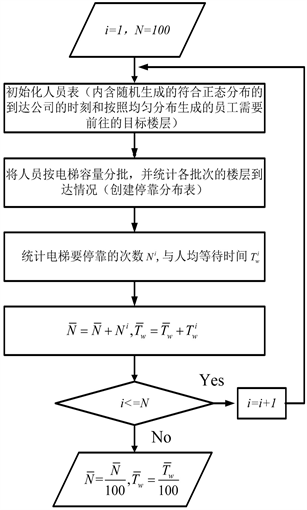Figure 6. Overall flow chart of simulation and statistical calculation of average waiting time and stopping number

6.3.2. 高峰时期电梯运行流程模型

6.3.1中我们已经给出平均等待时间 ${\stackrel{¯}{T}}_{w}$ 与停靠次数 ${\stackrel{¯}{N}}_{}$ 仿真统计计算整体流程图，但对每一个仿真方案的具体每一次仿真的停靠次数 ${N}^{i}$ 与平均等待时间 ${T}_{w}^{i}$ 还未给出具体细节的讨论。

Step 1：初始化得到本轮高峰所有750个人的到达时刻，以及每个人的期望到达楼层列表，并按照到达时间T的以及期望到达楼层L，按照到达时间的前后排序，早到在前晚到在后，并设定ABCD电梯的当前可用时间为 $Ta,Tb,Tc,Td=0$

Step 2：对时间为前i位的电梯乘客，的期望到达楼层Li进行判断其属于AC电梯还是BD电梯，所属于AC电梯进入Step 3，若属于BD电梯则进入Step 4；

Step 3：若AC电梯的当前可用时间 $Ta\le Tc$ ，则当前第当i位电梯乘客进入A电梯，此时若A电梯达到承载率 20×μ 人则A电梯出发，更新A电梯的当前可用时间Ta，并计录A电梯此次的停靠次数Na；若 $Ta>Td$ ，则当前第当i位电梯乘客进入C电梯，此时若C电梯达到承载率 15×μ 人则C电梯出发，更新C电梯的当前可用时间Tc；并计录C电梯此次的停靠次数Nc；

Step 4：若BD电梯的当前可用时间 $Tb\le Td$ ，则当前第当i位电梯乘客进入B电梯，此时若B电梯达到承载率 $\text{2}0×\mu$ 人则B电梯出发，更新B电梯的当前可用时间Tb，并计录B电梯此次的停靠次数Nb；若 $Tb>Td$ ，则当前第当i位电梯乘客进入D电梯，此时若D电梯达到承载率 $15×\mu$ 人则D电梯出发，更新D电梯的当前可用时间Td，并计录D电梯此次的停靠次数Nd；

Step 5：若 $i=i+1$ ，若 $i\le 750$ 返回Step 2；

Step 6：根据Step 2~4过程，对A，B，C，D的所有批次运输电梯的每一个人的等待时间，和每次电梯出发的停靠的次数，统计算出第i伦高峰期间电梯停靠次数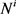与平均等待时间 ${T}_{w}^{i}$

$Tx={T}_{End}+\left({L}_{End}-1\right)×2+N×\left(8+2\right)+\left(N+1\right)$

${T}_{End}$ ：该电梯当前批次的最终出发时间，也就是当前批次最后一个人的到达时间；

${L}_{End}$ ：该电梯当前批次的最大出发楼层，也就是电梯需要运行的最高位置，到达后即返回；

$N$ ：该电梯当前批次的停靠次数。

6.3.3. 模型求解结果Table 5. Simulation results of candidate solutions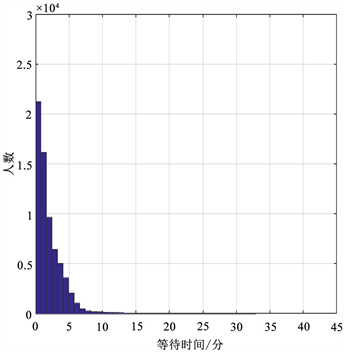Figure 7. Waiting time-population distribution map

6.4. 问题3——电梯调度方案设计(含地下停车库)

6.4.1. 电梯运行流程模型(含地下停车库)

$Tx={T}_{End}+\left({L}_{End}-1\right)×2+N×\left(8+2\right)+\left(N+1\right)+F_delay$ ，其中 $x\in \left\{a,b,c,d\right\}$ ，上式中各项的意义如下

${T}_{End}$ ：该电梯当前批次的最终出发时间，也就是当前批次最后一个人的到达时间；

${L}_{End}$ ：该电梯当前批次的最大出发楼层，也就是电梯需要运行的最高位置，到达后即返回；

$N$ ：该电梯当前批次的停靠次数。

6.4.2. 模拟计算结果Table 7. Simulation results of candidate solutions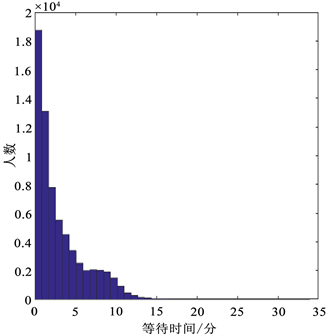Figure 8. Waiting time-population distribution map (South Korean elevator)

7. 模型的评价与改进

7.1. 模型的评价

1) 全文的模型求解都运用了计算机模拟，使求解更接近现实，方案的评价及确定更加简单和合理；

2) 在问题二中，采用计算机仿真方法，实现对候选方案的重要指标的估计，缩小了模型的范围，而且在列举出的较优方案中直接对其模拟，易于求得最优方案；

3) 在问题三中，利用前面的结果加以适当调整，通过模拟得出最优方案，简单易行。

7.2. 模型的改进

1) 由于模型比较理想化，和现实中差距较大，我们的模型还存在不足之处。若要模型更加接近现实，更加精确，则必须重新考虑，放弃一些理想化的假设；

2) 在求解问题二和问题三的运行方案时，要使结果更加精准，必需建立统一的优化模型，或者将所有可列结果进行模拟，而不是在所给出的有限的几种方案中模拟，从中选取最优解。

8. 结束语

Computer Simulation and Optimization Design of Elevator Operation Plan[J]. 建模与仿真, 2018, 07(04): 209-222. https://doi.org/10.12677/MOS.2018.74025

1. 1. 韩中庚. 数学建模方法及其应用[M]. 第二版. 北京: 高等教育出版社, 2009.

2. 2. 张海龙, 高东红. 几种电梯运行模式的比较及应用[J]. 数学的实践与认识, 2008(10): 65-69.

3. 3. 李庆民, 刘君, 张志华, 武器系统仿真模型的可信性验证方法研究[J]. 系统仿真学报, 2006(12).